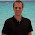## Wednesday, June 24, 2009

### Subclassing JSONEncoder and JSONDecoder

Today I was trying to write code to add the ability to encode datetime and timedelta objects as JSON. The idea is that two python applications would talk to eachother over an http request, with json as the content. It seemed simple, but I didn't realize that the decoder works a bit differently, and so it'll probably make you rethink how you write the encoder.

The json documentation shows an example of overriding the JSONEncoder:

`class ComplexEncoder(json.JSONEncoder):    def default(self, obj):        if isinstance(obj, complex):            return [obj.real, obj.imag]        return json.JSONEncoder.default(self, obj)`

Using this, I wrote my own encoder for datetimes...

`class DateTimeAwareJSONEncoder(JSONEncoder):    """     Converts a python object, where datetime and timedelta objects are converted    into strings that can be decoded using the DateTimeAwareJSONDecoder.    """    def default(self, obj):        if isinstance(obj, datetime):            return obj.strftime('dt(%Y-%m-%dT%H:%M:%SZ)')        elif isinstance(obj, timedelta):            days = obj.days            seconds = obj.seconds            milliseconds = obj.microseconds / 1000            milliseconds += obj.seconds * 1000            milliseconds += obj.days * 24 * 60 * 60 * 1000            return 'td(%d)' % (milliseconds)        else:            return JSONEncoder.default(self, obj)`

The default method is called with every object encountered, so whenever it finds a datetime or timedelta, it uses my custom code to convert it into a string that I should be able to pick out using a regular expression or some other means when decoding. Here's what I tried...

`datetime_regex = re.compile('\"dt\((\d{4})-(\d{2})-(\d{2})T(\d{2}):(\d{2}):(\d{2})Z\)\"')timedelta_regex = re.compile('\"td\((\d+)\)\"')class DateTimeAwareJSONDecoder(JSONDecoder):    """     Converts a json string, where datetime and timedelta objects were converted    into strings using the DateTimeAwareJSONEncoder, into a python object.    """    def decode(self, obj):        dt_result = datetime_regex.match(obj)         if dt_result:            year, month, day, hour, minute, second = map(lambda x: int(x), dt_result.groups())            return datetime(year, month, day, hour, minute, second)                td_result = timedelta_regex.match(obj)        if td_result:            milliseconds = int(td_result.groups())            return timedelta(milliseconds=milliseconds)        return super(DateTimeAwareJSONDecoder, self).decode(obj)`

This seemed to work, and it would so long as the data I input was just the datetime object...

`decoder = DateTimeAwareJSONDecoder()# Workeddecoder.decode('"dt(2009-04-01T23:51:23Z)"') # Didn't workdecoder.decode('["dt(2009-04-01T23:51:23Z)"]') decoder.decode('{"a": "dt(2009-04-01T23:51:23Z)"}')`

It turns out that the "decode" method on the JSONDecoder does not work like the "default" method on the JSONEncoder. As soon as I passed the call to the JSONDecoder's "decode" method, it went ahead and decoded the whole thing before passing it back.

For awhile I was still pretty sure this is how I should do it, and I just wasn't using the "decode" method right, until I read an article about the json encoder and decoder by Doug Hellmann. Here he showed how you could create a decoder a bit differently. The code looks as such (some changed to make it more readable):

`class MyDecoder(json.JSONDecoder):    def __init__(self):        json.JSONDecoder.__init__(self, object_hook=self.dict_to_object)    def dict_to_object(self, d):        if '__class__' in d:            class_name = d.pop('__class__')            module_name = d.pop('__module__')            module = __import__(module_name)            class_ = getattr(module, class_name)            args = dict( (key.encode('ascii'), value) for key, value in d.items())            inst = class_(**args)        else:            inst = d        return inst`

The important thing to see here is that rather than overriding a method, you're actually passing a method as a parameter to the parent constructor. This dict_to_object works a bit more like the "default" method, in that whenever you get an object this method is called to give you a chance to convert it. What's important to realize, however, is that it will only get called if the object in question is a json object. This sounded as if that would be okay with what I have, but remember that in json, the word "object" has a specific meaning. A string value is not an object, it's a string value. As such, it will get decoded from the json string to a python string, and your object hook method will not get to touch it.

The solution, then, is to drop the idea of converting the datetime and timedelta objects into strings, and instead convert them into objects. Here is the code...

`class DateTimeAwareJSONEncoder(JSONEncoder):    """     Converts a python object, where datetime and timedelta objects are converted    into objects that can be decoded using the DateTimeAwareJSONDecoder.    """    def default(self, obj):        if isinstance(obj, datetime):            return {                '__type__' : 'datetime',                'year' : obj.year,                'month' : obj.month,                'day' : obj.day,                'hour' : obj.hour,                'minute' : obj.minute,                'second' : obj.second,                'microsecond' : obj.microsecond,            }           elif isinstance(obj, timedelta):            return {                '__type__' : 'timedelta',                'days' : obj.days,                'seconds' : obj.seconds,                'microseconds' : obj.microseconds,            }           else:            return JSONEncoder.default(self, obj)class DateTimeAwareJSONDecoder(JSONDecoder):    """     Converts a json string, where datetime and timedelta objects were converted    into objects using the DateTimeAwareJSONEncoder, back into a python object.    """    def __init__(self):            JSONDecoder.__init__(self, object_hook=self.dict_to_object)    def dict_to_object(self, d):         if '__type__' not in d:            return d        type = d.pop('__type__')        if type == 'datetime':            return datetime(**d)        elif type == 'timedelta':            return timedelta(**d)        else:            # Oops... better put this back together.            d['__type__'] = type            return d`

I convert the datetime and timedelta to objects, using the '__type__' attribute so that I can pick them out of a crowd more easily. Doing it this way makes a lot more sense than going with strings, regular expressions, and the such.

1.Excellent example! I had to modify the JSONDecoder.__init__ call to pass extra keyword arguments.

def __init__(self,*args,**kargs):
JSONDecoder.__init__(self, object_hook=self.dict_to_object,*args,**kargs)

1.Thank you!
We do need extra keyword arguments for passing 'encoding'...

2.thanks for this

3.I passed your code through pylint and realize that to be strict the decoder init method should be like this:

def __init__(self):
json.JSONDecoder.__init__(super(DateTimeAwareJSONDecoder, self), object_hook=self.dict_to_object)

otherwise it complains that it is expecting JSONDecoder but receiving a DateTimeAwareJSONDecoder

1.isn't it better to use super() for that?

def __init__(self, *args, **kwargs):
super().__init__(self, object_hook=self.dict_to_object, *args, **kwargs)

4.What about timezone aware datetimes? And dates?

5.You should use jsonpickle for that.

1.jsonpickle provides a method for encoding python objects to string and back. It works out of the box for any type without the need to extend JSONEncoder and Decoder. Thanks!